## Posts

Showing posts from December 18, 2022

### Creating Pascal's Triangle in C#: for computer science at GCSE, IGCE, A Level and programmers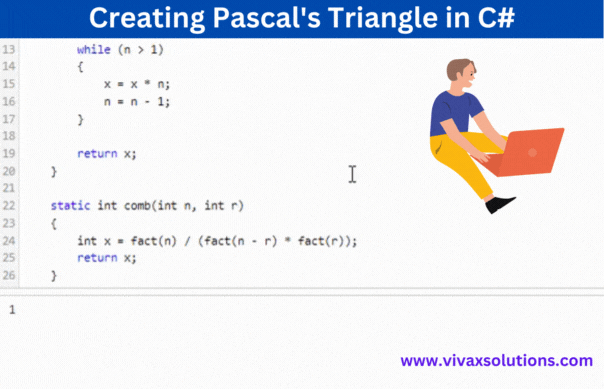Creation of Pascal's Triangle in C# The above animation is produced in C# programming language for students who do computer science at GCSE, IGCSE, IB and A Level. The code snippet consists of four major function that collectively produce the Pascal's Triangle. They are: A factorial function A function to generate combinations A function to generate Pascal's Triangle in the correct format The main function to run the function in step 3). The code snippet is given below; you can practise it interactively by providing the function, Pascal's Triangle(n) , the parameter required - the number of rows. Since the formatting is programmed up to 10 rows, please use a number below 10.

### Straight Line Graph Generator: for Year 9, GCSE Maths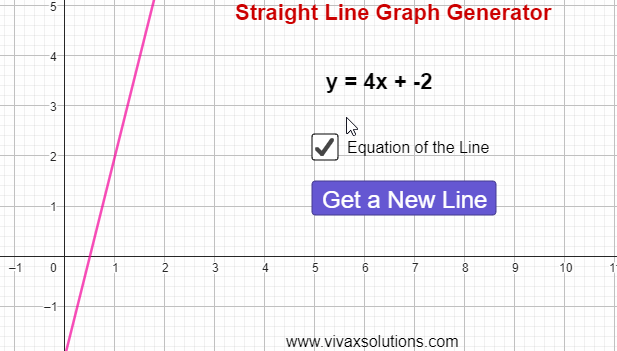The equation of a straight line is given by the formula, y = mx + c, where m and c are the gradient and intercept respectively. The gradient, m, is the slope or steepness of the line; the intercept, c, is the point where it crosses the y-axis at. E.g. A straight line passes through (1,2) and (3, 8). Find its equation. m = (8-2)/(3-1) = 3 m = 3 x = 1 y = 2 y = mx + c 2 = 3(1) + c c =  -1 The equation of the straight line is y = 3x - 1. Using the following simulation, you can create lines at random, calculate the equation as above and then check the answer, in order to be highly competent in the topic.

### Constructions for Maths: GCSE, IGCSE and GCE - OL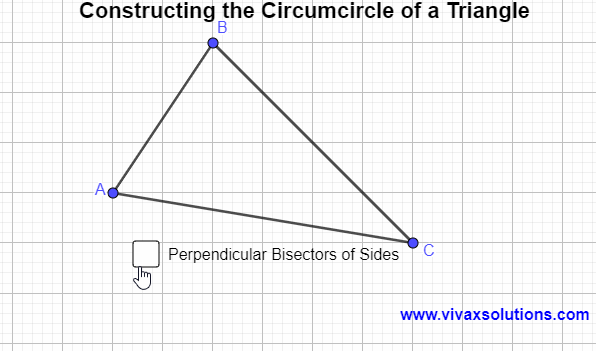A circumcircle is a circle that touches the three vertices of a circle from outside. It can easily be constructed by the following steps: Construct the perpendicular bisectors of each side of the triangle Locate the point of intersection of the bisectors The point of intersection is the centre of the circumcircle Construct the circle with centre in step 3). It is the circumcircle of the triangle in question.

### Correlation and the Line of Best Fit - for GCSE, IGCSE and A-Level Maths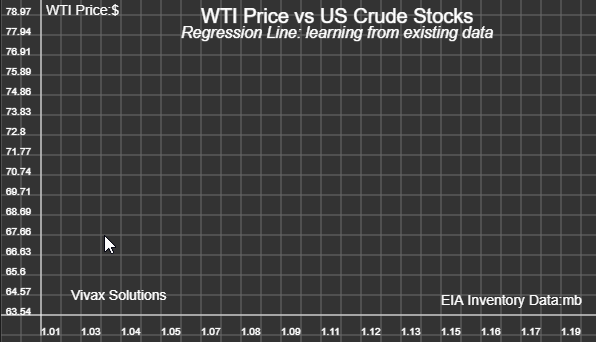The above animation shows how a code snippet written in JavaScript determines the line of best fit for an evolving set of data. The line is drawn accurately while performing the mathematical requirements for it to be accurate and minimizing the known errors. This is a stepping stone to AI - Artificial Intelligence - that can be best described as the ML - machine learning. In this case, the machine is learning from its own experience in plotting the dots on the grid. All you have to do is clicking at random points on the grid; the sketching the line of best is the responsibility of the programme. You can practise and have fun on your own here: A mild introduction to Machine Learning

### Solving equations by iteration - GCSE, IGCSE and A Level maths - using Python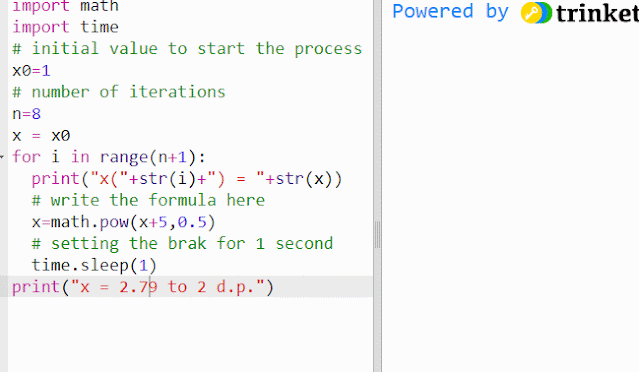Iteration If f(x) =0, it can be rearranged in the form of x = g(x), where both f(x) and g(x) are functions of x. Then x = g(x) is said to be in iterative form. E.g. x² - x - 5 = 0 x² = x + 5 x n+1 = √(x n + 5) - f(x) = 0 in iterative form If x n is known, x n+1 can be calculated. The above is not the only iterative form, though: two more iterative forms from can be derived from it; every form, however, may not produce the required result! it's important to choose the correct form. In order to start the process, the initial value, x 0 , is usually given. When the values of x becomes almost constant, the last value can be approximated as the solution or the root of the equation, to an appropriate significant level. For example, the above simulation shows how the process can be triggered off by taking x 0 = 1.  You can practise it here interactively; just change the value of x 0 and run the simulation:

### How to measure atmospheric pressure by a mercury barometer - a toxic legacy!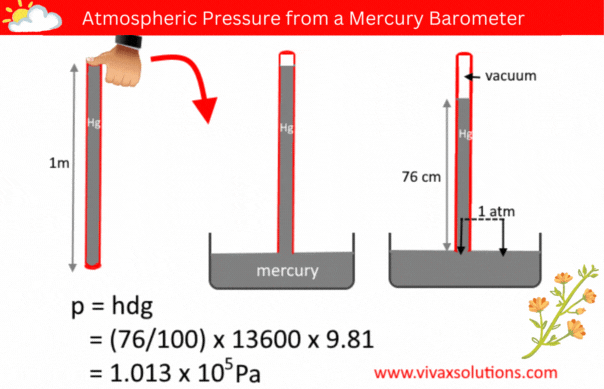The pressure of a liquid column is given by the formula, p = hdg, where h, d and g are the depth/height, density and the acceleration due to gravity respectively.  A few decades ago, in order to measure the atmospheric pressure, a simple barometer was used. It consisted of a thin, long, glass tube - sealed at one end - a laboratory stand with a clamp to hold it and a container full of mercury. The glass tube was usually a meter in length. In a school lab, a technician normally used to fill it with mercury to the brim, place his thumb at the open end, before inverting it and placed in the mercury container, while holding the tube vertically with the aid of the stand and clamp.   When left for a while for mercury to settle down, the technician measure the height of the mercury column. If the location in question is at a level closer to the sea level, the height of the mercury column is usually 76 cm. Since the pressure of a liquid at the same level is equal, the above diagram shows that